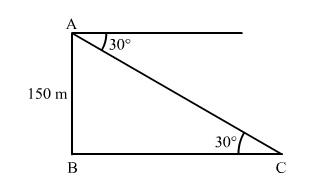# Choose the correct answer of the following question:`
Question:

Choose the correct answer of the following question:

The angle of depression of a car parked on the road from the top of a 150-m-high tower is 30°. The distance of the car from the tower is

(a) $50 \sqrt{3} \mathrm{~m}$

(b) $150 \sqrt{3} \mathrm{~m}$

(c) $150 \sqrt{2} \mathrm{~m}$

(d) $75 \mathrm{~m}$

Solution:Let AB be the tower and point C be the position of the car.

We have,

$\mathrm{AB}=150 \mathrm{~m}$ and $\angle \mathrm{ACB}=30^{\circ}$

In $\Delta \mathrm{ABC}$,

$\tan 30^{\circ}=\frac{\mathrm{AB}}{\mathrm{BC}}$

$\Rightarrow \frac{1}{\sqrt{3}}=\frac{150}{\mathrm{BC}}$

$\therefore \mathrm{BC}=150 \sqrt{3} \mathrm{~m}$

Hence, the correct answer is option (b)